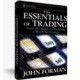Categories

Interest Rates and Stock Prices

After last week’s run up in interest rates, it’s worth taking some time to understand the link between rates and stock prices.

A stock’s price is based on two primary things. One is the value of its assets. That could be things like cash and other investments, real estate and gold reserves. Book value is often cited as a measure of these sorts of items, but not a great one given accounting methods.

The second source of stock pricing is earnings, specifically future earnings. A great deal of time and effort is spent forecasting those future earnings in order to determine what a stock is worth.

Here’s where the interest rate comes in.

The value of \$1 in a year is not the same as \$1 today. Why? Because if you have \$1 today you could invest it and earn a rate of return that would leave you with more than \$1 in a year’s time. For example, at 10% if you invest \$1 today you will end up with \$1.10 in a year. As a result of this, we need to discount the value of the \$1 received in a year to figure out how much we would invest today to get that amount.

To do so we use the interest rate. The higher the rate, the lower the value of \$1 earned a year from now in present terms (present value). For example, at 10% the value of \$1 received in one year is \$0.91. At 5%, the present value of that same \$1 is a bit over \$0.95.

In order to approximate the value of a stock, analysts take forecasts of future earnings – usually 5 five years – and discount those figures back to see what they are worth today. Since the value of future earnings decreases as interest rates increase (and as you move further out in time), so too does the value of the stock in question.

Here’s an example. Assume Company X is expected to make \$1 this year, \$2 next year, \$3 the year after, and so on out to \$5 in year 5. Using a 10% interest rate, the value of those payments is \$10.65. If we were to use a 5% rate, though, it would be \$12.57. (You can calcuate the above figures using the NPV function in Excel.)

So where does the rate that we use come from? Well, in classical financial terms it would be the so called risk free rate. That’s the interest rate we could earn taking almost no risk of default on our investment. For all practical purposes, the risk free rate is normall the sovereign debt rate, such as that for the US Treasury secturity for the length of time in question. There are other alternatives, of course, but that really doesn’t matter much for this discussion. The point is that as interest rates change, they impact stock prices through this valuation process..By John

Author of The Essentials of Trading